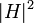# Minimal normal subgroup with order not dividing index is characteristic

## Statement

A minimal normal subgroup of a finite group whose order does not divide its index is characteristic.

## Facts used

1. Automorphisms preserve the property of being normal and hence of being minimal normal.
2. Normality is strongly intersection-closed
3. Product formula

## Proof

Given: A finite group$G$, a minimal normal subgroup$H$ of$G$. The order of$H$ does not divide the index$[G:H]$ of$H$ in$G$. An automorphism$\sigma$ of$G$.

To prove:$\sigma(H) = H$.

Proof:

Step no. Assertion/construction Facts used Given data used Previous steps used Explanation
1$\sigma(H)$ is a minimal normal subgroup of$G$. Fact (1)$\sigma$ is an automorphism and$H$ is a minimal normal subgroup of$G$. Given-cum-fact direct.
2$\sigma(H) \cap H$ is a normal subgroup of$G$. Fact (2) Step (1)$H$ is normal and$\sigma(H)$ is also normal by Step (1). Thus, by Fact (2),$\sigma(H) \cap H$ is normal.
3 Either$\sigma(H) \cap H$ is trivial, or$H = \sigma(H)$. Steps (1), (2) By Step (2),$\sigma(H) \cap H$ is normal. Since$H$ is minimal normal, the normal subgroup$\sigma(H) \cap H$ is either trivial or equals$H$. Similarly, since$\sigma(H)$ is minimal normal, the normal subgroup$\sigma(H) \cap H$ is either trivial or equals$\sigma(H)$. Combining these, we get the conclusion.
4 If$\sigma(H) \cap H$ is trivial, then$\langle H, \sigma(H) \rangle = H\sigma(H)$ is a normal subgroup of$G$ of order$|H|^2$. Fact (3) Step (1) By Step (1),$\sigma(H)$ is normal. So is$H$. Thus, their product is their join. If$H \cap \sigma(H)$ is trivial, the product has order$|H||\sigma(H)|$ by Fact (3), which simplifies to$|H|^2$ because$|H| = |\sigma(H)|$.
5$|H|^2$ does not divide$|G|$. Fact (4)$|H|$ does not divide$[G:H]$. By Lagrange's theorem,$[G:H] = |G|/|H|$.$|H|$ dividing$|G|/|H|$ is equivalent to$|H|^2$ dividing$|G|$.
6$\sigma(H) \cap H$ is nontrivial. Fact (4) Steps (4), (5) If$\sigma(H) \cap H$ is trivial, then Step (4) gives us a subgroup of$G$ of order$|H|^2$. By Fact (4), this would give that$|H|^2$ divides$|G|$. However, by Step (5),$|H|^2$ does not divide$|G|$.
7$H = \sigma(H)$. Steps (3) and (6) Step-combination direct.